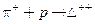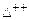Chapter 33, Problem 23PE

Chapter
Section
Textbook Problem

The reaction(described in the preceding problem) takes place via the strong force. (a) What is the baryon number of theparticle?(b) Draw a Feynman diagram of the reaction showing the individual quarks involved.

To determine

(a)

The baryon number of Δ++ particle.

Explanation

Given:

Reaction given, π++pΔ++

Calculation:

Baryon number of π+ particle is 0 and that of proton is +1.

For the given reaction,π++pΔ++

B:  0  +1

Therefore, to conserve baryon number for the above strong reaction, the baryon number

of Δ++ particle should be equal to +1

To determine

(b)

To draw Feynman diagram for the reaction π++pΔ++.

Still sussing out bartleby?

Check out a sample textbook solution.

See a sample solution

The Solution to Your Study Problems

Bartleby provides explanations to thousands of textbook problems written by our experts, many with advanced degrees!

Get Started

Find more solutions based on key concepts